• 今天小编就为大家分享一篇Python 余弦相似度与皮尔逊相关系数 计算实例，具有很好的参考价值，希望对大家有所帮助。一起跟随小编过来看看吧
• 实现了计算Pearson相关，内含一个实例，详细的使用说明，和Pearson相关基础知识的介绍PPT
• 要理解 Pearson 相关系数，首先要理解协方差（Covariance）。协方差表示两个变量 X，Y 间相互关系的数字特征，其计算公式为： 当 Y = X 时，即与方差相同。当变量 X，Y 的变化趋势一致时，如果某个 Xi 大于 X¯，...
一、理论知识：

要理解 Pearson 相关系数，首先要理解协方差（Covariance）。协方差表示两个变量 X，Y 间相互关系的数字特征，其计算公式为：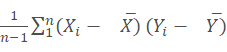当 Y = X 时，即与方差相同。当变量 X，Y 的变化趋势一致时，如果某个 Xi 大于 X¯，相应的 Yi 也大于 Y¯；如果某个 Xi小于 X¯，相应的 Yi 也小于¯Y¯，那么 COV(X,Y)COV(X,Y) 就是正值，当变量 X，Y 的变化趋势相反时，那么 COV(X,Y)COV(X,Y) 就是负值。

Pearson 相关系数公式如下：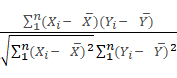由公式可知，Pearson 相关系数是用协方差除以两个变量的标准差得到的，虽然协方差能反映两个随机变量的相关程度（协方差大于0的时候表示两者正相关，小于0的时候表示两者负相关），但其数值上受量纲的影响很大，不能简单地从协方差的数值大小给出变量相关程度的判断。为了消除这种量纲的影响，于是就有了相关系数的概念。
当相关系数为1时，成为完全正相关；当相关系数为-1时，成为完全负相关；相关系数的绝对值越大，相关性越强；相关系数越接近于0，相关度越弱。
二、函数：Pearsonr（x,y）
1）输入：x为特征，y为目标变量.
2）输出：r： 相关系数 [-1，1]之间，p-value: p值。
注： p值越小，表示相关系数越显著，一般p值在500个样本以上时有较高的可靠性。
三、python实现Pearson correlation coefficient
############导包#################
import numpy as np
from scipy.stats import pearsonr
import csv
############导入数据#############
############切分数据#############
X = Housing_dataset[:,1:-1]
y = Housing_dataset[:,-1:]
print(X.shape)
print(y.shape)
#############Pearsonr分析#########
for i in range(0,13,1):
x = X[:,i].reshape(-1,1)
print(x.shape)
#------------pearsonr（x,y）-------------
result = pearsonr(x,y)
#------------保存结果--------------------
with open('./dataset/Pearsonr_dataset03.csv','a+',encoding='GB18030',newline="")as file_write:
result_writer = csv.writer(file_write)
result_writer.writerow(result)
Reference：
https://blog.csdn.net/huangfei711/article/details/78456165
https://blog.csdn.net/zhouwenyuan1015/article/details/65938847
展开全文相关性分析
• Python实现统计描述以及计算皮尔逊相关系数


文章目录
（一）、数据示例（二）、从excel文件中读取数据（三）、统计描述（四）、将统计描述输出到excel文件中（五）、综合代码（六）、结果展示

关于Python数据分析在数学建模中的更多相关应用：Python数据分析在数学建模中的应用汇总（持续更新中！）

（一）、数据示例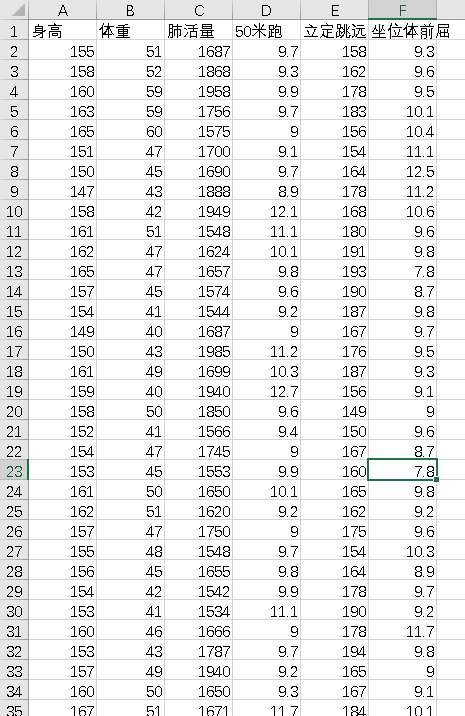（二）、从excel文件中读取数据
#从excel文件中读取数据
wb = xlrd.open_workbook(filename=file)#打开文件
sheet = wb.sheet_by_index(0)#通过索引获取表格
rows = sheet.nrows # 获取行数
all_content = []        #存放读取的数据
for j in range(0, 6):       #取第1~第6列对的数据
temp = []
for i in range(1,rows) :
cell = sheet.cell_value(i, j)   #获取数据
temp.append(cell)
all_content.append(temp)    #按列添加到结果集中
temp = []
return np.array(all_content)

（三）、统计描述
#统计描述
def calculate(datas):
MIN = np.min(datas,axis = 1)    #计算最小值
MAX = np.max(datas,axis = 1)    #计算最大值
AVG = np.average(datas,axis = 1)    #计算平均值
MEDIAN = np.median(datas,axis = 1)  #计算中位数
SKEWNESS =stats.skew(datas,axis = 1)    #计算偏度
KURTOSIS = stats.kurtosis(datas,axis = 1)   #计算峰度
STD = np.std(datas,axis = 1)    #计算标准差
result = np.array([MIN,MAX,AVG,MEDIAN,SKEWNESS,KURTOSIS,STD])   #形成一个矩阵
return result

（四）、将统计描述输出到excel文件中
#将统计描述输出到excel文件中
writer = pd.ExcelWriter('C:\\Users\\lenovo\\Desktop\\数学建模\\相关系数\\A.xlsx')		# 写入Excel文件
writer.save()
writer.close()

（五）、综合代码
# -*- coding: utf-8 -*-
"""
Created on Mon Jul 29 16:53:19 2019

@author: lenovo
"""
import xlrd
import numpy as np
import pandas as pd
from scipy import stats
from scipy.stats import pearsonr

#从excel文件中读取数据
wb = xlrd.open_workbook(filename=file)#打开文件
sheet = wb.sheet_by_index(0)#通过索引获取表格
rows = sheet.nrows # 获取行数
all_content = []        #存放读取的数据
for j in range(0, 6):       #取第1~第6列对的数据
temp = []
for i in range(1,rows) :
cell = sheet.cell_value(i, j)   #获取数据
temp.append(cell)
all_content.append(temp)    #按列添加到结果集中
temp = []
return np.array(all_content)

#统计描述
def calculate(datas):
MIN = np.min(datas,axis = 1)    #计算最小值
MAX = np.max(datas,axis = 1)    #计算最大值
AVG = np.average(datas,axis = 1)    #计算平均值
MEDIAN = np.median(datas,axis = 1)  #计算中位数
SKEWNESS =stats.skew(datas,axis = 1)    #计算偏度
KURTOSIS = stats.kurtosis(datas,axis = 1)   #计算峰度
STD = np.std(datas,axis = 1)    #计算标准差
result = np.array([MIN,MAX,AVG,MEDIAN,SKEWNESS,KURTOSIS,STD])   #形成一个矩阵
return result

#将统计描述输出到excel文件中
writer = pd.ExcelWriter('C:\\Users\\lenovo\\Desktop\\数学建模\\相关系数\\A.xlsx')		# 写入Excel文件
writer.save()
writer.close()

result = calculate(datas)   #统计描述
corrcoe = np.corrcoef(result)   #计算皮尔逊相关系数


（六）、结果展示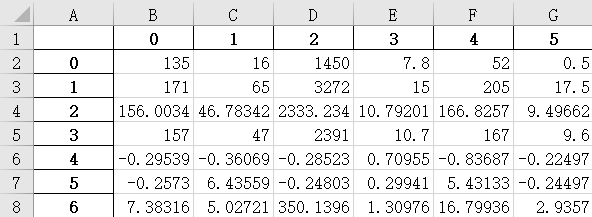展开全文• 先定义欧几里得距离函数;先定义皮尔逊系数函数，利用for循环把皮尔逊系数公式的各个组成部分算出来，计算皮尔逊系数，调用函数计算皮尔逊系数PYHON
• 皮尔逊相关系数 定义 皮尔逊相关系数判断两组数据与某一直线拟合程度的一种度量 公式及其计算过程如下 python代码实现 ''' @copyright Programming Collective Intelligence 返回p1和p2...
皮尔逊相关系数
定义

皮尔逊相关系数判断两组数据与某一直线拟合程度的一种度量  公式及其计算过程如下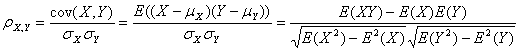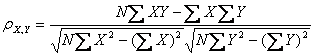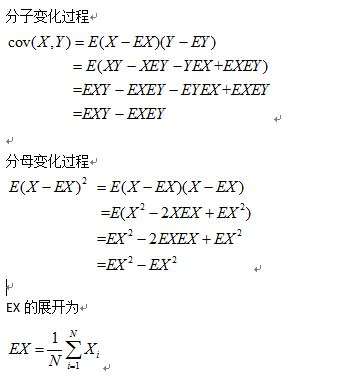python代码实现
'''
返回p1和p2的皮尔逊相关系数
prefs是一个二维矩阵字典，如下图所示
（名字，电影）  movie1   movie2
p1      4.5      5.0
p2      3.0      1.5
'''
def simPearson(prefs, p1, p2):
#得到双方都曾评价过得物品列表
si={}
for item in prefs[p1]:
for item in prefs[p2]:
si[item] = 1

#得到列表元素的个数
n = len(si)

#如果两者没有共同之处，则返回1
if n == 0: return 1

#对所有偏好求和
sum1 = sum([prefs[p1][it] for it in si])
sum2 = sum([prefs[p2][it] for it in si])

#求平方和
sum1Sq = sum([pow(prefs[p1][it], 2) for it in si])
sum2Sq = sum([pow(prefs[p2][it], 2) for it in si])

#求乘积之和
pSum = sum([prefs[p1][it] * prefs[p2][it] for it in si])

#计算皮尔逊评价值
num = pSum - (sum1 * sum2) / n
den = sqrt((sum1Sq-pow(sum1, 2) / n) * (sum2Sq - pow(sum2, 2) / n))

if den == 0: return 0

r = num / den

return r
展开全文相关性系数
• / n) #计算皮尔逊相关系数 den = sqrt((sumofx2 - float (sum1 ** 2 ) / n) * (sumofy2 - float (sum2 ** 2 ) / n)) return num / den a = [3.3, 6.5, 2.8, 3.4, 5.5] b = [3.5, 5.8, 3.1, 3.6, 5.1] c = [5.6, ...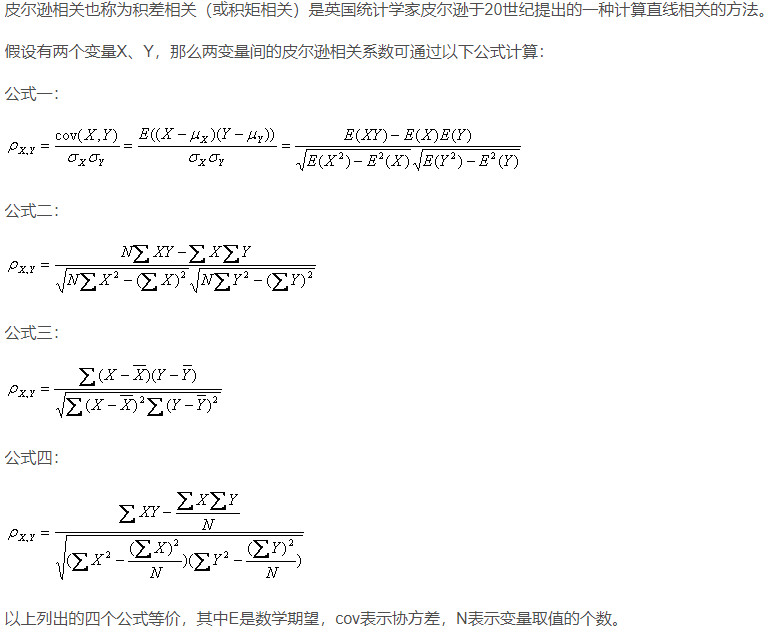评分矩阵如下表所示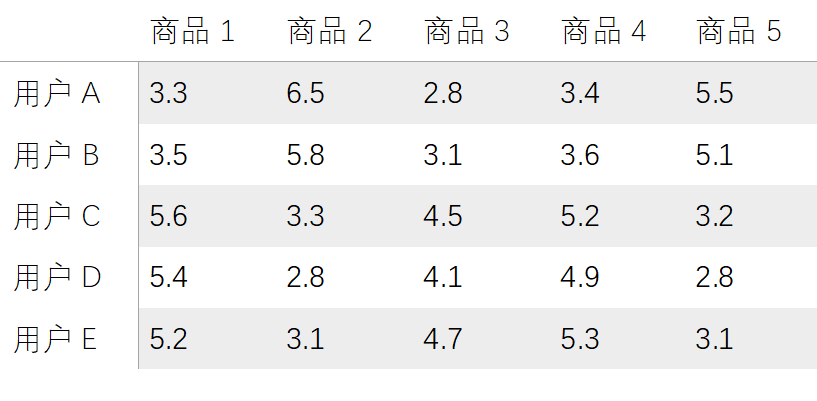from  math  import  sqrt

def  multipl(a,b):
sumofab = 0.0
for  i  in  range ( len (a)):
temp = a[i] * b[i]
sumofab += temp
return  sumofab

def  corrcoef(x,y):
n = len (x)
#求和
sum1 = sum (x)
sum2 = sum (y)
#求乘积之和
sumofxy = multipl(x,y)
#求平方和
sumofx2  =  sum ([ pow (i, 2 )  for  i  in  x])
sumofy2  =  sum ([ pow (j, 2 )  for  j  in  y])
num = sumofxy - ( float (sum1) * float (sum2) / n)
#计算皮尔逊相关系数
den = sqrt((sumofx2 - float (sum1 ** 2 ) / n) * (sumofy2 - float (sum2 ** 2 ) / n))
return  num / den

a = [3.3, 6.5, 2.8, 3.4, 5.5]
b = [3.5, 5.8, 3.1, 3.6, 5.1]
c = [5.6, 3.3, 4.5, 5.2, 3.2]
d = [5.4, 2.8, 4.1, 4.9, 2.8]
e = [5.2, 3.1, 4.7, 5.3, 3.1]

print(corrcoef(a,b))
print(corrcoef(a,c))
print(corrcoef(a,d))
print(corrcoef(a,e))
print(corrcoef(b,c))
print(corrcoef(b,d))
print(corrcoef(b,e))
print(corrcoef(c,d))
print(corrcoef(c,e))
print(corrcoef(d,e))


结果为：

             相关系数

用户A&B   0.9997735176536731
用户A&C  -0.8477583108627492
用户A&D  -0.8418164002035229
用户A&E  -0.9152367092225393
用户B&C  -0.8417411591004959
用户B&D  -0.835319954072308
用户B&E  -0.9099753379926111
用户C&D   0.9989872824995625
用户C&E   0.9762719892992101
用户D&E   0.9697821610909036


展开全文• 0 皮尔逊系数 1 python计算方法 1.1 根据公式手写 1.2 numpy的函数 1.3 scipy.stats中的函数 0 皮尔逊系数  在统计学中，皮尔逊相关系数( Pearson correlation coefficient），又称皮尔逊积矩相关系数（Pearson ...
• 文章目录0 皮尔逊系数1 python计算方法1.1 根据公式手写1.2 numpy的函数1.3 scipy.stats中的函数 0 皮尔逊系数  在统计学中，皮尔逊相关系数( Pearson correlation coefficient），又称皮尔逊积矩相关系数（Pearson...
• 在研究脑网络时，看到了用皮尔逊相关系数计算脑功能网络的，就简单的学了一下它的计算 这里用到的是Python的numpy库 首先，最前面要加import，先导入这个模块 import numpy as np 然后，调用corrcoef() 其中data...numpy
• 皮尔逊相关系数的计算(python代码版) 1 2 3 4 5 6 7 8 9 10 11 12 13 14 15 16 17 18 19 20 21 22 23 24 25 ...算法 C 数据结构 matlab
• python 加opencv计算两张图像的hu矩和皮尔逊相关系数，输出其相似度。 import cv2 import math import numpy as np from PIL import Image im1 = cv2.imread('xuetaggujia.png',cv2.IMREAD_GRAYSCALE) im2 =...opencv
• from scipy.stats import pearsonr x = [0.5, 0.4, 0.6, 0.3, 0.6, 0.2, 0.7, 0.5] y = [0.6, 0.4, 0.4, 0.3, 0.7, 0.2, 0.5, 0.6] print(pearsonr(x, y)) ...# r:相关系数[-1，1]之间 # p:p值越小  ...
• 查了一下，发现可以通过皮尔逊相关系数和spearman相关系数来计算特征之间的相似度，下面将介绍这两个系数和区别。 皮尔逊相关系数 下面是皮尔逊相关系数的计算公式，只需要将（X和Y的协方差）/（X的标准差*Y的标准...特征工程 机器学习
• 在统计学中，皮尔逊相关系数( Pearson correlation coefficient），又称皮尔逊积矩相关系数（Pearson product-moment correlation coefficient，简称 PPMCC或PCCs）。用于衡量两个变量X和Y之间的线性相关相关关系，...特征选择
• python计算皮尔逊pearson相关系数|普通最小二乘法（OLS）进行多项式拟合统计学 机器学习 数据挖掘
• 皮尔逊相关系数具有平移不变性和尺度不变性，计算出了两个向量（维度）的相关性。  在python中的实现：' import numpy as np x=np.random.random(10) y=np.random.random(10) #方法一：根据公式求解 x_=x-...
• 数据探索 计算相关系数 为了更加准确地描述变量之间的线性相关...皮尔逊相关系数（Pearson Correlation Coefficient） 一般用于分析两个连续性变量之间的关系，其计算公式如下。 r=∑i=1n(xi−x‾)(yi−y‾)∑i=1n(x...
• python程序计算皮尔逊相关系数、最大信息系数以及灰色关联度
• 基于皮尔逊相关系数的用户相似推荐算法python实现 随着电子商务规模的不断扩大，商品个数和种类快速增长，顾客需要花费大量的时间才能找到自己想买的商品。这种浏览大量无关的信息和产品过程无疑会使淹没在信息过载...推荐系统
• 皮尔逊系数 在统计学中，皮尔逊相关系数( Pearson correlation coefficient），又称皮尔逊积矩相关系数（Pearson product-moment correlation coefficient，简称 PPMCC或PCCs）。用于衡量两个变量X和Y之间的线性相关...1024程序员节
• pearson相似度的计算公式： 其中是均值，是指 x 的均值。 代码如下所示： def mean_processed(a): b = np.zeros_like(a) num = 0 for i,j in enumerate(a): if j != 0: ... b[i] = ...
• Python Implementation # Input: 2 objects # Output: Pearson Correlation Score def pearson_correlation(object1, object2): values = range(len(object1)) # Summation over all attributes for both ......

# 皮尔逊相关系数pythonpython 订阅